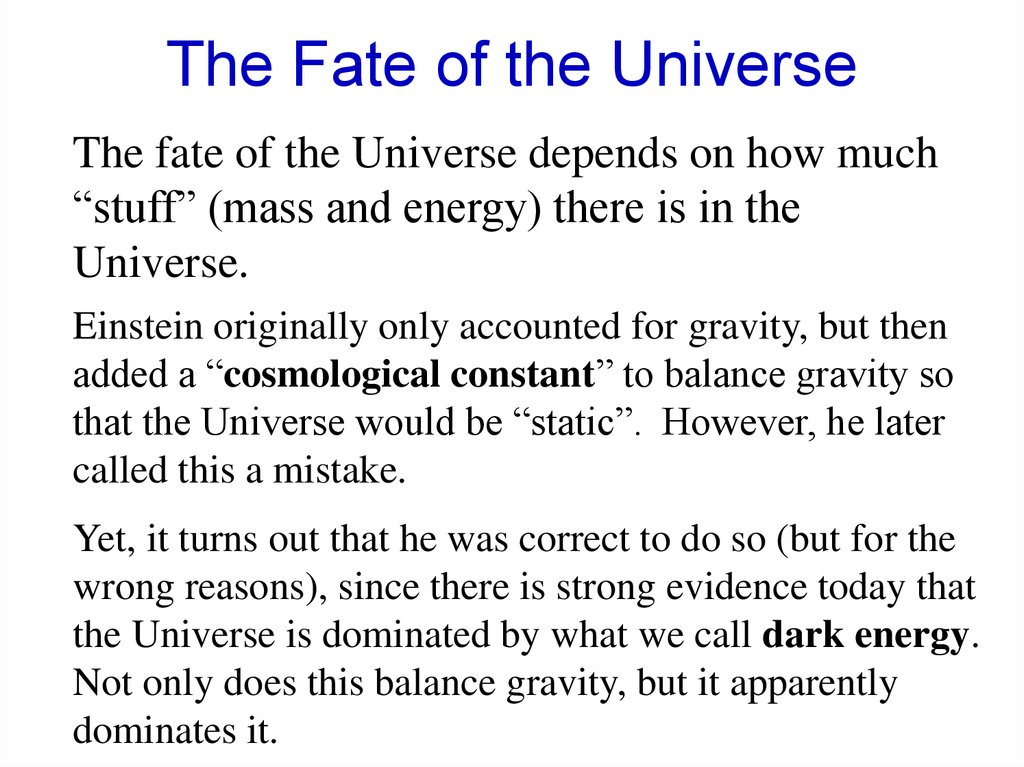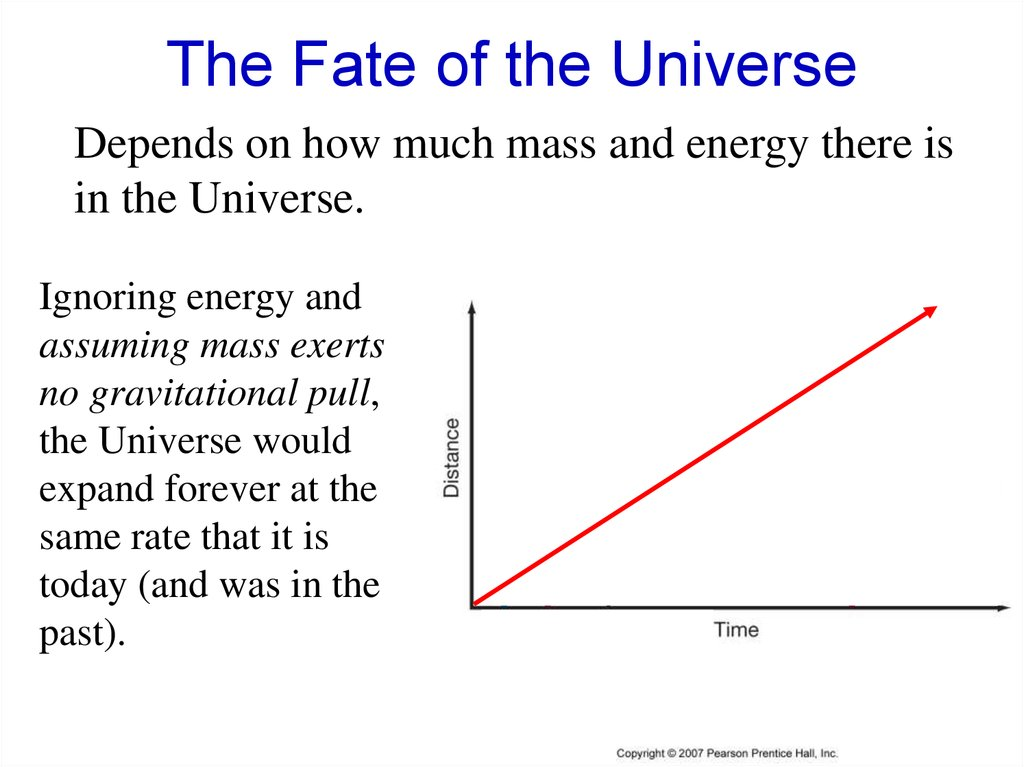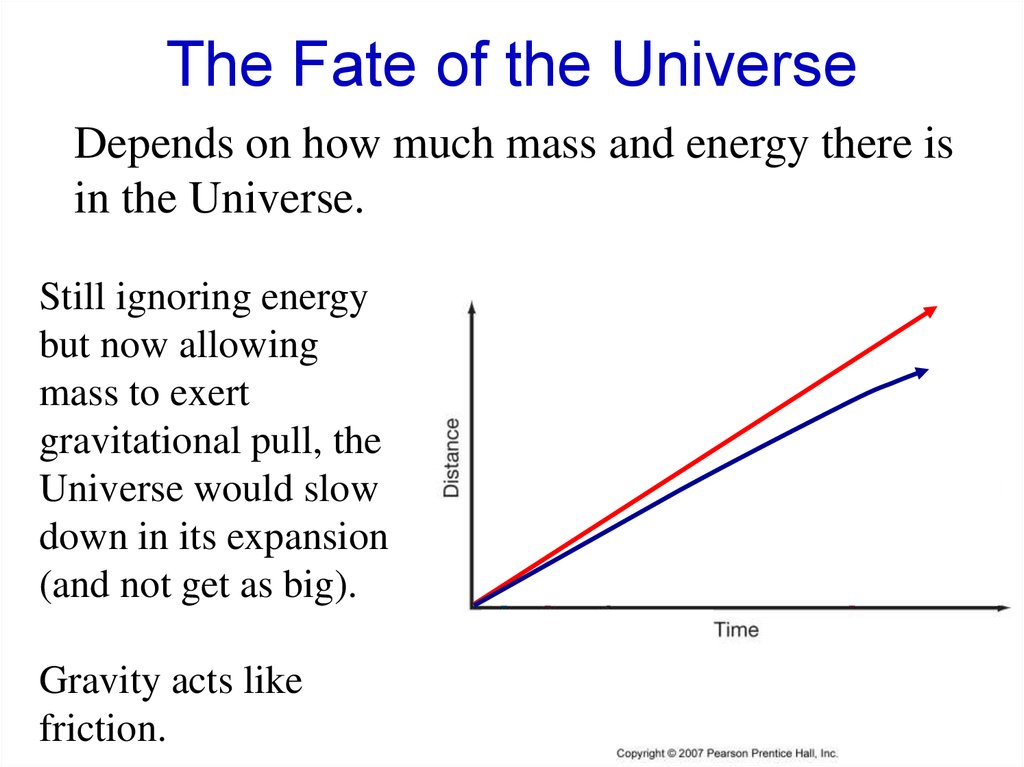# The fate of the universe

## 1. The Fate of the Universe

The fate of the Universe depends on how much
“stuff” (mass and energy) there is in the
Universe.
Einstein originally only accounted for gravity, but then
added a “cosmological constant” to balance gravity so
that the Universe would be “static”. However, he later
called this a mistake.
Yet, it turns out that he was correct to do so (but for the
wrong reasons), since there is strong evidence today that
the Universe is dominated by what we call dark energy.
Not only does this balance gravity, but it apparently
dominates it.

## 2. The Fate of the Universe

Depends on how much mass and energy there is
in the Universe.
Ignoring energy and
assuming mass exerts
no gravitational pull,
the Universe would
expand forever at the
same rate that it is
today (and was in the
past).

## 3. The Fate of the Universe

Depends on how much mass and energy there is
in the Universe.
Still ignoring energy
but now allowing
mass to exert
gravitational pull, the
Universe would slow
down in its expansion
(and not get as big).
Gravity acts like
friction.

## 4. The Fate of the Universe

Depends on how much mass and energy there is
in the Universe.
If there is enough
mass the Universe
will collapse back
upon itself.

## 6. The Fate of the Universe

Depends on how much mass and energy there is
in the Universe.
But Dark Energy has
a repulsive force that
can cause the
expansion of the
Universe to speed up
again.

## 7. The Fate of the Universe

Depends on how much mass and energy there is
in the Universe.
More matter (or less
Dark Energy) would
could the reexpansion to happen
later.

## 8. Age of the Universe

In reality, the
different curves
should meet
today rather than
at the Big Bang.
This has
important
implications for
the age of the
Universe.
Possible explanation for the acceleration:
vacuum pressure (cosmological constant),
more generically called dark energy.

## 9. An Accelerating Universe?

Type I supernovae can be used to measure the
behavior of distant galaxies.
In a decelerating Universe, we expect to see
more distant galaxies receeding relatively faster
than nearby galaxies.

## 10.

Where we expected the data to be
Where it really is.
However, when we
look at the data, we
see that it corresponds
not to a decelerating
universe, but to an
accelerating one.
That is, the Universe is
dominated by dark
energy.

## 11. The Critical Density

The amount of mass needed to just barely
make the Universe closed is called the
critical density ( c) (ignoring the effects of
dark energy).
Astronomers like to talk about the density of the
Universe in terms of the ratio of the real density
to the critical density ( ).
c

## 12. Density and the Fate of the Universe

If > 1, the Universe will eventually
collapse.
If < 1, the Universe will expand
forever.
If = 1, the Universe just barely
manages to expand forever.

## 13. Density

If the density is low, the universe will expand
forever.
If it is high, the
universe will
ultimately
collapse.

## 14. Fate of the Cosmos

The answer to this question lies in the
actual density of the Universe.
Measurements of luminous matter
suggest that the actual density is only a
few percent of the critical density.
But – we know there must be large
amounts of dark matter.

## 15.

However, the best estimates for the amount of
dark matter needed to bind galaxies in clusters,
still only bring the observed density up to about
0.3 times the critical density, and it seems very
unlikely that there could be enough dark matter
to make the density critical.
We can test this by
measuring the
distances and
redshifts of objects.

## 16. Dark Energy and The Cosmological Constant

Curiously, Einstein had introduced this idea
decades before in order to balance gravity and
make the Universe “static”.
He later called it the biggest blunder of his career.
Turns out he was right.